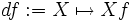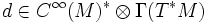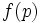# De Rham derivative of a function

## Definition

### Definition with symbols

Let$M$ be a differential manifold. The de Rham derivative is an operator that takes as input an infinitely differentiable function on$M$, and outputs a differential 1-form on$M$. It is defined as follows:$df := X \mapsto Xf$

In other words, the de Rham derivative of a function$f$ sends a vector field$X$ to the function$Xf$.

## Facts

### Where it lives

The de Rham derivative can be viewed as:$d:C^\infty(M) \to \Gamma(T^*M)$

i.e. it is a map from the algebra of infinitely differentiable functions, to the space of differential 1-forms.

It can also be viewed as an element:$d \in C^\infty(M)^* \otimes \Gamma(T^*M)$

### Not tensorial

However, the de Rham derivative is not tensorial. In other words,$df$ at a point$p \in M$ cannot be determined from$f(p)$.

### Leibniz rule

Further information: de Rham derivative satisfies Leibniz rule

The de Rham derivative satisfies the Leibniz rule, namely:$d(fg) = f(dg) + g(df)$

This is a direct consequence of the Leibniz rule for derivations.

## Related notions

### Exact 1-form

Further information: Exact 1-form

An exact 1-form is a differential 1-form, or a section of the cotangent bundle, that can be expressed as the de Rham derivative of a function.

### de Rham derivative of a differential 1-form

Further information: de Rham derivative of a differential 1-form

There is a closely related notion called the de Rham derivative of a differential 1-form.